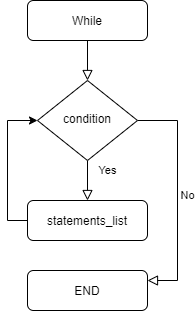Open in App
Not now

# MySQL WHILE Loop

• Difficulty Level : Basic
• Last Updated : 21 Jan, 2022

In this, we will cover the overview of MySQL WHILE Loop and then will cover the algorithm of each example and then will see the analysis of each example. Let’s discuss it one by one.

Introduction :
MySQL WHILE loop statement is used to execute one or more statements again and again, as long as a condition is true. We can use the loop when we need to execute the task with repetition while condition is true.
Note –
Use a WHILE LOOP statement in case you are unsure of what number of times you would like the loop body to execute. Since the WHILE condition is evaluated before entering the loop, it’s possible that the loop body might not execute even once.

Syntax :

```[label_name:] WHILE
condition DO
statements_list
END WHILE [label_name]```

Syntax label meaning –

• Label_name –
label_name is optional, it’s a name related to the WHILE loop.
• Condition –
condition is tested each undergoes through the WHILE loop. If the condition results in TRUE, the statements_list is executed, or if the condition results in FALSE, the WHILE loop is terminated.
• Statements_list –
Statements_list is that the list of statements to be executed withstand the WHILE loop.

Block diagram of While loop :Block diagram of WHILE loop

Examples of MySQL WHILE Loop :

Example-1 :
Lets us create a function using a while loop.

```DELIMITER \$\$
CREATE FUNCTION GeekInc ( value INT )
RETURNS INT
BEGIN
DECLARE inc INT;
SET inc = 0;
label:
WHILE inc <= 30000 DO
SET inc = inc + value;
END
WHILE label;
RETURN inc;
END; \$\$
DELIMITER ;```

Analysis –

• Value is the input for the GeekInc function.
• inc is declared and set to 0.
• While inc is less than and equal to 3000, it will set inc to inc + value.

To check output used the following command given below.

`CALL GeekInc(10000);`

Output –

`0, 10000, 20000, 30000`

Example-2 :
Let us create a procedure using a while loop.

```DELIMITER \$\$
CREATE procedure while_ex()
block: BEGIN
declare value VARCHAR(20) default ' ' ;
declare num INT default 0;
SET num = 1;
WHILE num <= 5 DO
SET value = CONCAT(value, num ,',' );
SET num = num + 1;
END
WHILE block;
select value ;
END \$\$
DELIMITER ;```

Analysis –

• create procedure while_ex and declare value and num.
• set num at 1, while num is equal to or less than 5 do
• set value equal to concatenation of value and num.

To check output used the following command given below.

`call while_ex();`

Output –

Example-3 :
Let us create a table “Test_Cal” which has dates as follows.

```CREATE TABLE Test_Cal(
t_in INT AUTO_INCREMENT,
fulldate DATE UNIQUE,
day TINYINT NOT NULL,
month TINYINT NOT NULL,
PRIMARY KEY(id)
);```

Now, create a stored procedure to insert data into the table as follows.

```DELIMITER \$\$
CREATE PROCEDURE InsertCal(dt DATE)
BEGIN
INSERT INTO Test_Cal(
fulldate,
day,
month )
VALUES(dt,
EXTRACT(DAY FROM dt),
EXTRACT(MONTH FROM dt)
);
END\$\$
DELIMITER ;```

Now create stored procedure LoadCal() that updates the number of days starting from a start date into the table.

```DELIMITER \$\$
startDate DATE,
day INT
)
BEGIN
DECLARE counter INT DEFAULT 1;
DECLARE dt DATE DEFAULT startDate;
WHILE counter <= day DO
CALL InsertCal(dt);
SET counter = counter + 1;
SET dt = DATE_ADD(dt,INTERVAL 1 day);
END WHILE;
END\$\$
DELIMITER ;```

Analysis –

• The stored procedure LoadCal() has two parameters: startDate and day.
• First, declare a counter and dt variables for saving values.
• Then, check if the counter is less than or equal day, if yes:
• Run stored procedure Inertial() to insert a row into the Test_Cal table.
• An increase in counter by 1 increases the dt by 1 day using the DATE_ADD().
• The WHILE loop inserts date into the table till the counter is the same as the day.

To check output used the following command given below.

```CALL LoadCal('2021-01-01',31);
select * from Test_Cal where tid < 10 ;```

Output –

My Personal Notes arrow_drop_up
Related Articles